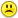+0

# (T*(cos(45)/cos(30)))*sin(30) + (T*sin(45) - 50 = 0 What is T and how to solve?

0
948
6

(T*(cos(45)/cos(30)))*sin(30) + (T*sin(45) - 50 = 0 What is T and how to solve?

Apr 12, 2014

#1
0

$$T\times\frac{cos45}{cos30}\times sin30+Tsin45-50&=0\\\\ T\left(\frac{1}{\sqrt{2}}\div\frac{\sqrt3}{2}\right)\frac{1}{2}+T\times\frac{1}{\sqrt2}&=50\\\\ T\left(\frac{1}{\sqrt{2}}\times\frac{2}{\sqrt3}\times\frac{1}{2}\right)+\frac{T}{\sqrt2}&=50\\\\ \frac{T}{\sqrt{6}}+\frac{T}{\sqrt2}=50\\\\ \sqrt{6}\times\left(\frac{T}{\sqrt{6}}+\frac{T}{\sqrt2}\right)=50\times\sqrt{6}\\\\ T+\sqrt{3}T=50\sqrt{6}\\\\ T(1+\sqrt{3})=50\sqrt{6}\\\\ T=\frac{50\sqrt{6}}{1+\sqrt{3}}\\\\ T=\frac{50\sqrt{6}}{1+\sqrt{3}}\times\frac{1-\sqrt3}{1-\sqrt{3}}\\\\ T=\frac{50\sqrt{6}-50\sqrt{18}}{1-3}\\\\ T=75\sqrt{2}-25\sqrt6$$

.
Apr 13, 2014
#2
0

Idk how to reply to anwser but i think you did it wrong melody, thanks for the help anyways but the anwser is 45 not 75*square root(2) - 50*square root (6). If you figure out where you went wrong though i would be glad to hear you explanation, you work was really helpful so thanks

Apr 13, 2014
#3
0

Hi,

I have changed my answer a little bit but I don't think that it is 45.

Other people will look at this thread so you will get more input.

I think mine is correct.

We will see what the others think.

Thanks for posting and for the feedback.

Melody

PS There was a bracket missing in the original question - is my interpretation of what you wanted correct?

Apr 13, 2014
#4
0

I think Melody made a slight error towards the end....I'm pretty sure the answer isn't EXACTLY 45, either.

(T*(cos(45)/cos(30)))*sin(30) + (T*sin(45) - 50 = 0 ???

When I stick "45" in for "T,"  we get

$$\left({\mathtt{45}}{\mathtt{\,\times\,}}\left({\frac{\underset{\,\,\,\,^{{360^\circ}}}{{cos}}{\left({\mathtt{45}}^\circ\right)}}{\underset{\,\,\,\,^{{360^\circ}}}{{cos}}{\left({\mathtt{30}}^\circ\right)}}}\right)\right){\mathtt{\,\times\,}}\underset{\,\,\,\,^{{360^\circ}}}{{sin}}{\left({\mathtt{30}}^\circ\right)}{\mathtt{\,\small\textbf+\,}}\left({\mathtt{45}}{\mathtt{\,\times\,}}\underset{\,\,\,\,^{{360^\circ}}}{{sin}}{\left({\mathtt{45}}^\circ\right)}{\mathtt{\,-\,}}{\mathtt{50}}\right) = {\mathtt{0.190\: \!978\: \!224\: \!309\: \!896\: \!5}}$$

Let's see what we can do....taking Melody's work from this point......

50√(6) / (1 + √(3))

Let's multiply by the whole fraction by the conjugate of (1 + √(3)) on "top" and "bottom"

We have

50√(6) / (1 + √(3))   *   (1 - √(3)) / (1 - √(3))

This gives us

50√(6) * (1 - √(3)) / (-2)

Sinplifying the top, we have

[50√(6)- 50√(18)] / (-2)

Let's multiply top and bottom by (-1) to get rid of that pesky (-2).....Nore that the things in the numerator just switch places when we do this...so we have

[50√(18)- 50√(6)] / (2)....and dividing everything by 2, we get

25 (√(18)- √(6)).....we could simplify the "18" under the square root, but we would still have two square roots, so let's just leave it alone.

So "T" = 25 (√(18)- √(6)) .......BTW this is close to 45 !!!!!

And plugging in for "T" we have

25*(√(18)-√(6))*(cos(45)/cos(30))*sin(30)+(25*(√(18)-√(6))*sin(45)-50)

If you evaluate this answer inyour calculator (or the calculator on this site), you'll get something that ≈ 0

(Sorry....they've made some changes to this site and I haven't figured out how to evaluate things, just yet)

Anyway.....I feel pretty confident that this is the correct answer

Apr 13, 2014
#5
0

thanks thata the anwser and youre right its not exaclty 45, im sorry if that was missleading.

Apr 13, 2014
#6
0

Melody here